# Solid State & Group Theory

## 20 Questions MCQ Test GATE Chemistry Mock Test Series | Solid State & Group Theory

Description
Attempt Solid State & Group Theory | 20 questions in 60 minutes | Mock test for Chemistry preparation | Free important questions MCQ to study GATE Chemistry Mock Test Series for Chemistry Exam | Download free PDF with solutions
QUESTION: 1

### Nickel forms a face centered cubic unit cell with a = 351.8 pm. The value of crystallographic radius of a nickel atom is

Solution: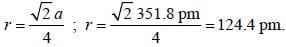QUESTION: 2

### The unit cell of an element of atomic mass 96 and density 10.3 g/cm–3 is a cube with edge length of 314 pm the structure of the crystal lattice is

Solution: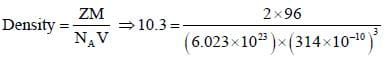The structure of solid should be bcc.

*Answer can only contain numeric values
QUESTION: 3

### Lithium boron hydride crystallizes in an orthorhombic system with 4  molecules per unit cell. The unit cell dimensions are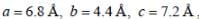If the molar mass is 21.76. The density of crystal is ______g/cm-3. (Round off to two decimal places).x1

Solution: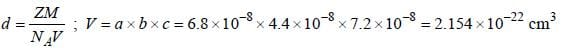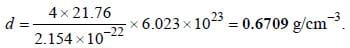*Answer can only contain numeric values
QUESTION: 4

A metal crystallizes in simple cubic unit cell. The length of the edge of the unit cell 'a' is 6.22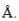The radius of each atom of the metal is ______() (Round of to two decimal places).

Solution:

For simple cubic structure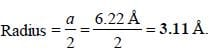QUESTION: 5

In n-type semi conductors the correct statement is/are
(i) The fermi energy level lies in between donor band and valence band
(ii) The fermi energy level lies in donor band and conduction band
(iii) The fermi energy increases with increasing temperature
(iv) The fermi energy decreases with increasing temperature

Solution: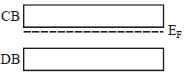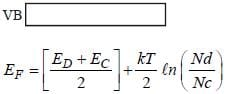QUESTION: 6

The diamond unit cell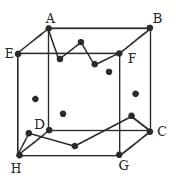can be representd as

Solution:

Corners are represented by 0, 1.
Face centres (left, right, top, bottom) by (½)
Face centres front and back by 0, 1
Td Vd centres by 1/4 and 3/4.

QUESTION: 7

In an ionic oxide, oxide ions are arranged in hcp array and positive ion occupy two thirds of octahedral void simplest formula of metal M is

Solution: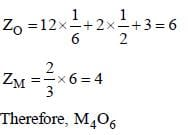QUESTION: 8

In case of Fullerene the correct relation between number of faces (F), number of vertices (V) and number of edges (E) is

Solution:

F + V = E + 2
Correct option is (d)

QUESTION: 9

The point group of Admantane is

Solution:

Tetrahedral (Td)
Correct option is (d)

QUESTION: 10

The point group of Bicyclo 1, 1, 1-pentane is

Solution:

Point group = D3h

*Answer can only contain numeric values
QUESTION: 11

The first order reflection of a beam of X-rays from a given crystal occur at 15o. The 3rd order reflection will occurs at angle of _____ degree. (Round off to two decimal places).

Solution: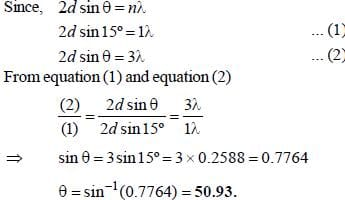*Answer can only contain numeric values
QUESTION: 12

A metal (atomic weight = 26.08), crystallizes in the cubic system with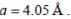Its density is 2.7g/cm-3. The radius of the atom of this metal will be _____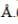(Round off to two decimal places).

Solution: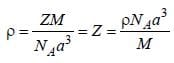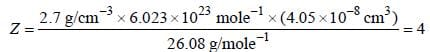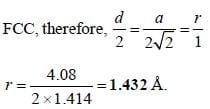*Answer can only contain numeric values
QUESTION: 13

The distance between the body-centered atom and one corner atom in sodium a =0.424 nm is ____nm. (Round off to two decimal places).

Solution:

The atom at the center of the cube is supposed to touch the two nearest corner atoms. The longest diagonal at which all these three atoms lie will be length 4r.
Therefore, distance between center atom and the corner atom = 2r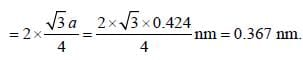*Answer can only contain numeric values
QUESTION: 14

The ionic radii of Cs+ and Cl-1 ions are 1.69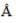and 1.81respectively. The co-ordinate number of Cs+ is _____(answer should be an integer).

Solution: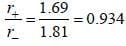As the radius ratio lies between 0.732 and 1.0 the co-ordinate number of Cs+ is 8.

*Answer can only contain numeric values
QUESTION: 15

For primitive cubic crystal with a = 3 x 1010m, the smallest diffraction angle θ, for (110) plane for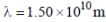is ______(Round off to two decimal places).

Solution: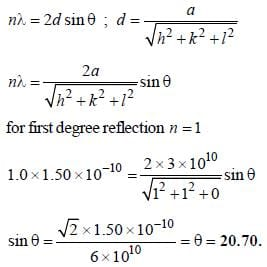QUESTION: 16

The first order diffraction of X-rays from a ceratin set of crystal planes occurs at an angle of 11.8º from the planes, if the planes are 0.281 nm a part the wavelength of X-rays is

Solution: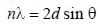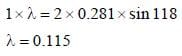QUESTION: 17

Match the crystal system unit cells mentioned in column-I with their characteristic feature mentioned in column-II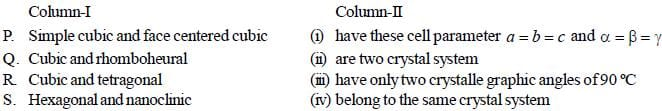Solution:
QUESTION: 18

The improper axis present in staggered ferrocene is

Solution:

The point group of ferrocene is D5d. It has S2n improper axis called S10.

QUESTION: 19

The Mulliken Notation for the following irreducible representation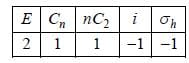Solution: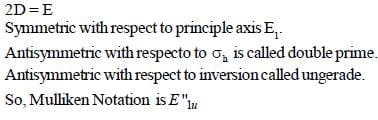*Answer can only contain numeric values
QUESTION: 20

The number of classes in CH4 molecule is/are ________(answer should be an integer).

Solution:

The number of classes in CH4 molecule is 5.Use Code STAYHOME200 and get INR 200 additional OFF Use Coupon Code